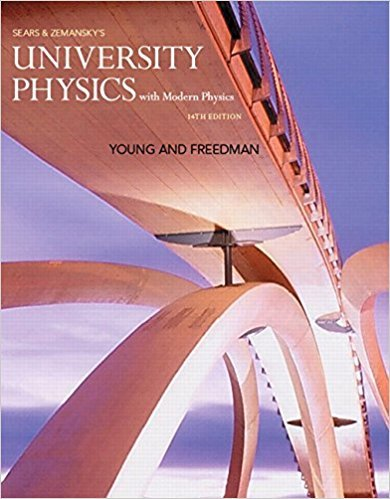×
×

# Answer: A Honda Civic travels in a straight line along aISBN: 9780321973610 228

## Solution for problem 2.6 Chapter 2

University Physics with Modern Physics (1) | 14th Edition

• Textbook Solutions
• 2901 Step-by-step solutions solved by professors and subject experts
• Get 24/7 help from StudySoup virtual teaching assistantsUniversity Physics with Modern Physics (1) | 14th Edition

4 5 1 323 Reviews
12
3
Problem 2.6

A Honda Civic travels in a straight line along a road. The cars distance x from a stop sign is given as a function of time t by the equation x1t2 = at 2 - bt 3 , where a = 1.50 m>s 2 and b = 0.0500 m>s 3 . Calculate the average velocity of the car for each time interval: (a) t = 0 to t = 2.00 s; (b) t = 0 to t = 4.00 s; (c) t = 2.00 s to t = 4.00 s.

Step-by-Step Solution:
Step 1 of 3
Step 2 of 3

Step 3 of 3

##### ISBN: 9780321973610

This textbook survival guide was created for the textbook: University Physics with Modern Physics (1), edition: 14. This full solution covers the following key subjects: . This expansive textbook survival guide covers 44 chapters, and 4574 solutions. The answer to “A Honda Civic travels in a straight line along a road. The cars distance x from a stop sign is given as a function of time t by the equation x1t2 = at 2 - bt 3 , where a = 1.50 m>s 2 and b = 0.0500 m>s 3 . Calculate the average velocity of the car for each time interval: (a) t = 0 to t = 2.00 s; (b) t = 0 to t = 4.00 s; (c) t = 2.00 s to t = 4.00 s.” is broken down into a number of easy to follow steps, and 90 words. The full step-by-step solution to problem: 2.6 from chapter: 2 was answered by , our top Physics solution expert on 01/09/18, 07:46PM. University Physics with Modern Physics (1) was written by and is associated to the ISBN: 9780321973610. Since the solution to 2.6 from 2 chapter was answered, more than 239 students have viewed the full step-by-step answer.

Unlock Textbook Solution